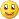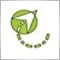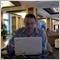# Stochastic Oscillator, the beginning - page 9232

i was interested if there are any stochastic indicators that can calculate what is the price price where two K% (or D%) of two Stochastics crossover.

thanks.3203

Scrat:
i was interested if there are any stochastic indicators that can calculate what is the price price where two K% (or D%) of two Stochastics crossover. thanks.

Melph's indi (prev.pages) shows 3(tree) stoch. crossover46

banzai:
Stoch (6,3,3) to Stoch (24,3,3). Place the template in the template folder and not in the indicator folder. Google for keywords: "Spuds Stochastic Thread Theory"

Has anyone tried to make an EA for the "Spuds Stochastic Threads", would that be too complex? Too bad the Spuds topic over at FF was not continued much further into this intriguing indicator...5

i have a new startey to make ea. who can built up togater with me the stochastic ea....that can follow up the market movement... i have the setting for stochastic and the agorithem for the stochastic that can be bulit....5921204Moderator
113455

I moved your post to this thread. Just hope that you will find something.3203

seems like you looking 4 alert once per bar after bar close?

(D1)9

Hull-Stochastic, anyone? Have a look!

Hi everyone,

This is my favorite oscillator and I can't find it in MQL4. I am hoping someone likes the look of it and will code it up for the TSD forum. I can do some Tradestation but no MQL4.

Pretty simple, it is Hull-Stochastic. A raw %k stochastic with Hull MA post-smoothing. I've never seen anyone use it before, I came up with it one night (not exactly rocket science, but it's nice). I have enclosed a pic of it and how I often use it. You can see it is pretty like a woman. Nice timely reversal points, nice and smooth, and it identifies trends very well with the longer Hull settings. It is so smooth I don't bother with a signal line, just the oscillator itself.

The pic shows the H-Stoch overlaid 3 times, with settings of (4,8) (30,40) and (40,50). Those numbers are in this order: (raw %k periods, Hull smoothing periods).

If anyone is interested here is the code. It is in an indicator and a function. I wrote the code for the 3 H-Stoch panel with the colors and I got the function code from the Tradestation forum. I appreciate any help and others might like this as well.

Cheers,

Scott

INDICATOR:

----------------------------------------------------------------------

Panel of 3 with Turning Point markers.}

{author: turboscottomatic}

{March 2007}

Inputs:

Stoch1Periods (4),

HMA1Periods (8),

Stoch2Periods (30),

HMA2Periods (40),

Stoch3Periods(50),

HMA3Periods(60),

ExtremeOverBought (100),

OverBought (80),

OverSold (20),

ExtremeOverSold (0);

Value1 = HullMA (fastk (Stoch1Periods), HMA1Periods);

Value2 = HullMA(fastk(Stoch2Periods), HMA2Periods);

Value3 = HullMA (fastk (Stoch3Periods), HMA3Periods);

Value4 = RGB(255, 128, 128); {Pink}

Value5 = RGB(0, 113, 0); {A nicer DarkGreen}

Value7 = RGB(31, 55, 224); {Little lighter Blue - still dark though}

Condition1 = Value1 >= Value1 and Value1 < Value1;

Condition2 = Value2 >= Value2 and Value2 < Value2;

Condition3 = Value3 >= Value3 and Value3 < Value3;

Condition4 = Value1 = Value1;

Condition5 = Value2 = Value2;

Condition6 = Value3 = Value3;

Plot1 (ExtremeOverBought, "ExtremeOB");

Plot2 (OverBought, "OverBought");

Plot3 (OverSold, "Oversold");

Plot4 (ExtremeOverSold, "ExtremeOS");

if Value3 >= Value3 then

begin

setplotcolor(5, green);

Plot5 (Value3, "HStoch3");

end

else if Value3 < Value3 then

begin

setplotcolor(5, Value5);

Plot5 (Value3, "HStoch3");

end;

if Value2 >= Value2 then

begin

setplotcolor(6, cyan);

Plot6 (Value2, "HStoch2");

end

else if Value2 < Value2 then

begin

setplotcolor(6, Value7);

Plot6 (Value2, "HStoch2");

end;

if Value1 >= Value1 then

begin

setplotcolor(7, Value4);

Plot7 (Value1, "HStoch1");

end

else if Value1 < Value1 then

begin

setplotcolor(7, red);

Plot7 (Value1, "HStoch1");

end;

Plot8(50, "MidLine");

FUNCTION:

------------------------------------------------------------------------

{From jtHMA - Hull Moving Average Function}

{Original Author: Atavachron, May 2005}

Inputs: price(NumericSeries), length(NumericSimple);

Vars: halvedLength(0), sqrRootLength(0);

{

Original equation is:

--------------------

waverage(2*waverage(close, period/2) - waverage(close, period), SquareRoot(period))

Implementation below is more efficient with lengthy Weighted Moving Averages.

In addition, the length needs to be converted to an integer value after it is halved and

its square root is obtained in order for this to work with Weighted Moving Averaging

}

if ((ceiling(length / 2) - (length / 2)) <= 0.5) then

halvedLength = ceiling(length / 2)

else

halvedLength = floor(length / 2);

if ((ceiling(SquareRoot(length)) - SquareRoot(length)) <= 0.5) then

sqrRootLength = ceiling(SquareRoot(length))

else

sqrRootLength = floor(SquareRoot(length));

Value1 = 2 * WAverage(price, halvedLength);

Value2 = Waverage(price, length);

Value3 = Waverage((Value1 - Value2), sqrRootLength);

HullMA = Value3;

Files:
h-stoch2.gif 179 kb8

Possible Stochastic Indicator???

If this has been posted before sorry. But, can't find this anywhere.

What I would like to see if an indicator with alarm sounding when the Stochastic crosses, up or down, exists. This is NOT an indicator of a trade entry for me just a HEAD'S UP that a POSSIBLE trade is coming. Other indicators (just 2) would complete a possible trade entry.

Also, would be nice if the Inputs on this indicator could be set, or changed.

Thanks!!